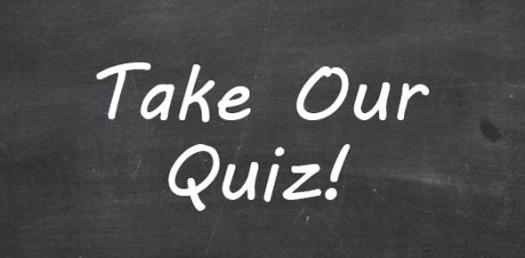# Mr. Thornburgh's Polygon Quiz

10 Questions | Total Attempts: 24Settings.

Related Topics
• 1.
How many sides does a Pentagon have?
• A.

6

• B.

5

• C.

10

• D.

7

• 2.
What is the name of a polygon with 4 Sides
• A.

Square

• B.

Octagon

• C.

• D.

Pentagon

• 3.
What is the least number of sides a polygon can have?
• A.

One

• B.

Two

• C.

Zero

• D.

Three

• 4.
How many angles does a Undecagon have?
• A.

11

• B.

12

• C.

10

• D.

9

• 5.
A stop sign is the shape of what polygon?
• A.

Hexagon

• B.

Octagon

• C.

Triangle

• D.

Heptagon

• 6.
What makes a regular polygon?
• A.

All sides the same length

• B.

All angles are congruent

• C.

All angles and sides are the same

• D.

All angles are under 90 degrees

• 7.
Which one of these is not a polygon?
• A.

Square

• B.

Triangle

• C.

Dodecagon

• D.

Circle

• 8.
All polygons must:
• A.

Have equal side lengths

• B.

All lines are connected

• C.

No curved lines

• D.

All the Above

• E.

Both B and C

• 9.
What type of polygon is this?
• A.

Pentagon

• B.

Square

• C.

• D.

Triangle

• 10.
Whats the name of this polygon?
• A.

Dodecagon

• B.

Nonagon

• C.

Pentagon

• D.

Hexagon